Search
• Madalina Ciortan

# Overview of feature selection methods

### Common strategies for choosing the most relevant features in your data set## The importance of feature selection

Selecting the right set of features to be used for data modelling has been shown to improve the performance of supervised and unsupervised learning, to reduce computational costs such as training time or required resources, in the case of high-dimensional input data to mitigate the curse of dimensionality. Computing and using feature importance scores is also an important step towards model interpret-ability.

## Overview of this post

This post shares the overview of supervised and unsupervised methods for performing feature selection I have acquired after researching the topic for a few days. For all depicted methods I also provide references to open-source python implementations I used in order to allow you to quickly test out the presented algorithms. However, this research domain is very abundant in terms of methods which have been proposed during the last 2 decades and as such this post only attempts to present my current limited view without any pretense for completeness. For a more comprehensive study, you can check the following review.

## Supervised/ Unsupervised models

There are supervised feature selection algorithms which identify the relevant features for best achieving the goal of the supervised model (e.g. a classification or a regression problem)and they rely on the availability of labelled data. However, for unlabeled data, a number of unsupervised feature selection methods have been developed which score all data dimensions based on various criteria, such as their variance, their entropy, their ability to preserve local similarity, etc. Relevant features identified using unsupervised heuristics can also be used in supervised models as they may discover other patterns in the data additional to the correlation of features with the target variable.

From a taxonomic point of view, feature selection methods usually fall into one of the following 4 categories detailed below: filter, wrapper, embedded and hybrid classes.

## Wrapper methods

This approach evaluates the performance of a subset of features based on the resulting performance of the applied learning algorithm (e.g. what is the gain in accuracy for a classification problem). Any learning algorithm can be used in this combination of search strategy and modelling.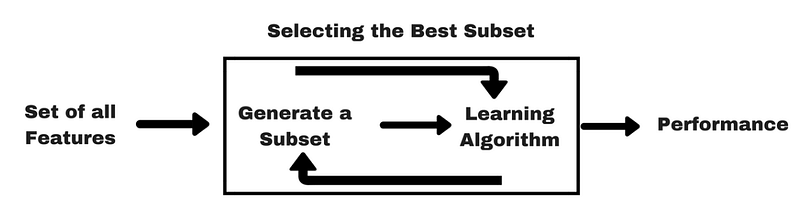Forward selection: this approach starts with an empty set of features and then the dimensions providing the best performance are being iteratively added to the result set

Backward selection: this approach starts from the set of all features and at each iteration the worst dimension is being removed

Implementation: these algorithms are implemented in the mlxtend package, find here an example of usage.

RFE (Recursive feature elimination): greedy search which selects features by recursively considering smaller and smaller sets of features. It ranks features based on the order of their elimination.

Implementation: scikit-learn

## Embedded methods

This approach consists in algorithms which simultaneously perform model fitting and feature selection. This is typically implemented by using a sparsity regularizer or constraint which makes the weight of some features become zero.

SMLR (Sparse Multinomial Logistic Regression): this algorithm implements a sparse regularization by ARD prior (Automatic relevance determination) for the classical multinational logistic regression. This regularization estimated the importance of each feature and prunes the dimensions which are not useful for the prediction.

Implementation: SMLR

ARD (Automatic Relevance Determination Regression): based on a Bayesian Ridge Regression, this model will shift the coefficient weights towards zero more than methods like OLS for instance.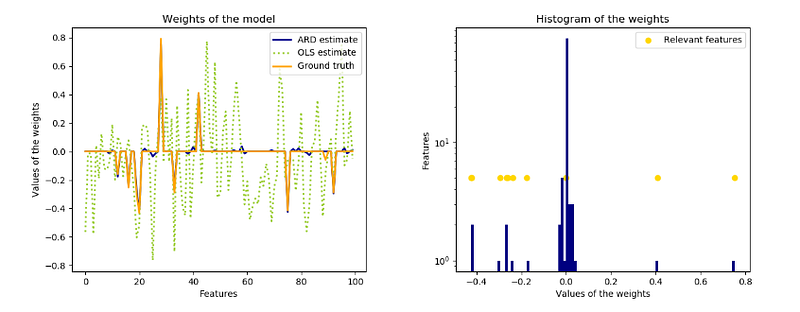Implementation: scikit-learn

Other examples of regularization algorithms: Lasso (implementing l1 regularization), Ridge Regression (implementing l2 regularization), Elastic Net (implementing l1 and l2 regularization). An intuitive depiction of these different regularization types shows that Lasso regression constrains the coefficients to a square shape, ridge creates a circle and elastic net is in between: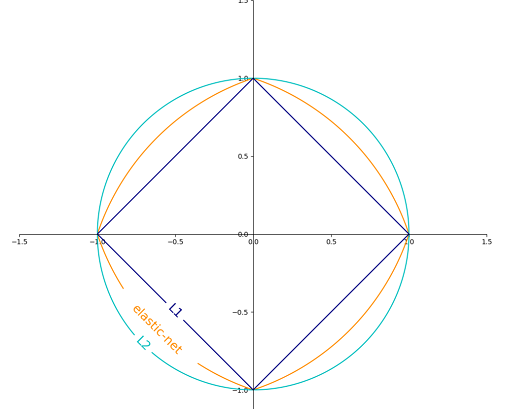A comprehensive description of these algorithms can be found here.

## Filter methods

This approach evaluates the importance of features based only on their inherent characteristics, without incorporating any learning algorithm. These methods tend to be faster and less computationally expensive than wrapper methods. If there is not enough data to model the statistical correlation between features, filter methods may provide worse results than wrapper methods. Unlike wrapper methods they are not subject to overfitting. They are extensively used on high dimensional data where wrapper methods have a prohibitive computational cost.

## Supervised methods

Relief : This method samples randomly instances from the dataset and updates the relevance of each feature based on the difference between the selected instance and the two nearest instances of the same and opposite classes. If a feature difference is observed in the neighboring instances of the same class ( a ‘hit’), the feature score decreases, alternatively if the feature value difference is observed with a different score (a ‘miss’) then the feature score increases.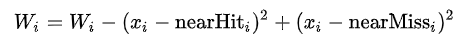The extended algorithm, ReliefF applies feature weighting and searches for more nearest neighbors.

Implementations: scikit-rebate, ReliefF

Fisher score: Typically used in binary classification problems, the Fisher ration (FiR) is defined as the distance between the sample means for each class per feature divided by their variances: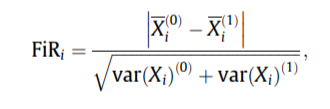Implementations : scikit-feature, usage example.

Chi-squared score:tests if there is a significant difference between the observed and the expected frequencies of 2 categorical variables. Thus the Null hypothesis states that there is no association between both variables.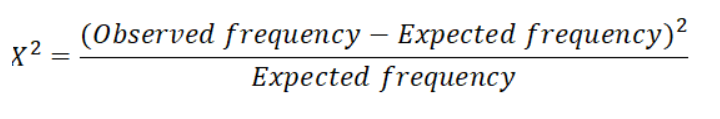In order to correctly apply the chi-squared in order to test the relation between various features in the dataset and the target variable, the following conditions have to be met: the variables have to be categorical, sampled independently and values should have an expected frequency greater than 5. The last condition ensures that the CDF of the test statistic can be closely approximated by the chi-square distribution, more details can be found here.

Implementation: sklearn, scipy

CFS (Correlation-based feature selection): The rationale of this method can be summarized as:

“ Features are relevant if their values vary systematically with category membership.”

Thus, good feature subsets contain features highly correlated with the classification and uncorrelated to each other. The method calculates the merit of a subset of k features as: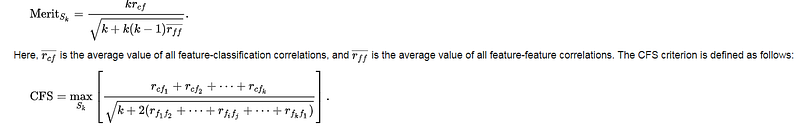Implementations : scikit-feature, usage example.

FCBF (Fast correlation-based filter): this method is faster and more efficient than both ReliefF and CFS and thus, more adapted for high dimensional input. In a nutshell, it follows a typical relevance-redundancy approach by computing first the Symmetrical Uncertainty (the information gain of x | y divided by the sum of their entropies) for all features, sorts them by this criteria and then removes the redundant features.

Implementations: skfeature, https://github.com/shiralkarprashant/FCBF

## Unsupervised methods

Variance: has been shown to be an effective way to select relevant features which tend to have a higher variance score

Implementation: Variance Threshold

Mean absolute difference: computes the mean absolute difference from the mean value (implementation).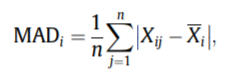Dispersion ratio: the arithmetic mean divided by the geometric mean. Higher dispersion corresponds to more relevant features (implementation)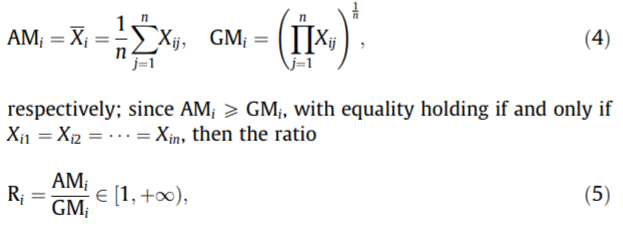Laplacian Score: is based on the observation that data from the same class is often close to each other and thus we can evaluate the importance of a feature by its power of locality preserving. The method consists in embedding the data on a nearest neighbor graph by using an arbitrary distance measure and then calculating a weight matrix. A laplacian score is then calculated for each feature and will have the property that smallest values correspond to the most important dimensions. However, in order to select a subset of features another clustering algorithm (e.g. k-means) is typically applied a-posteriori in order to select the best performing group

Implementations : scikit-feature

Laplacian Score combined with distance-based entropy: this algorithm builds on top of the Laplacian score and uses distance-based entropy to replace the typical k-means clustering and shows better stability in high-dimensional datasets (implementation)MCFS (Multi-Cluster Feature selection): a spectral analysis is performed to measure the correlation between different features. The top eigenvectors of the graph Laplacian are used to cluster the data and a feature score is being computed as explained in the original paper.

Implementation : https://github.com/danilkolikov/fsfc

LFSBSS (Localised feature selection), weighted k-means, SPEC and Apriori algorithms have been described in this review paper and are implemented in this package

## Hybrid methods

Another option of implementing feature selection consists in a hybrid approach of combining filter and wrapper methods into a 2 phase process: an initial filtering of features based on statistical properties (filter phase) followed by a second selection based wrapper methods.

## Other resources

There is a very abundant literature tackling feature selection problem and this post only scratches the surface of the research work which has been done. I will provide links to other resources that exist and that I haven’t yet tried.

A comprehensive list of other feature selection algorithms that I haven’t mentioned in this post have been implemented in scikit-feature package.

Other ways to identify relevant features is by using PLS (Partial least squares) as exemplified in this post or by performing linear dimensionality reduction techniques as presented here.

See All